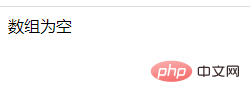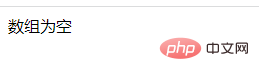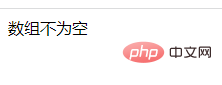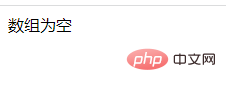# php怎么判断是否为空数组

3种判断方法：1、用count()或sizeof()计算数组的长度，判断数组长度是否为0，语法“count(数组)==0”，如果为0则为空数组，反之不为空数组。2、用empty()检查数组变量是否为空，语法“empty(数组)”，如果返回TRUE则是空数组。3、用“===”运算符判断数组是否为“[]”，语法“数组===[]”，如果返回TRUE则为空数组，反之则不为空数组。php判断一个数组是否为空数组的几种方法

`count(\$arr,\$m)`

\$m：为可选参数，可以省略。

• 如果省略 \$m 参数，或者将其设置为 COUNT_NORMAL 或者 0，count() 函数将不检测多维数组；

• 如果 \$m 设置为 COUNT_RECURSIVE 或者 1，count() 函数将递归计算数组中元素的个数，对于计算多维数组的元素个数尤为有用。

```<?php
// 声明一个空数组
\$empty_array = array();

// 检查数组是否为空
if(count(\$empty_array) == 0)
echo "数组为空";
else
echo "数组不为空";
?>```sizeof()函数是 count() 函数的别名，也就是所 sizeof() 函数的功能及使用方法与 count() 函数完全相同。

```<?php
// 声明一个空数组
\$empty_array = array();

if( sizeof(\$empty_array) == 0 )
echo "数组为空";
else
echo "数组不空";
?>```

empty() 函数用于检查一个变量是否为空。

empty() 判断一个变量是否被认为是空的。当一个变量并不存在，或者它的值等同于 FALSE，那么它会被认为不存在。如果变量不存在的话，empty()并不会产生警告。

```<?php
// 声明一个空数组
\$array = [];

// 检查数组是否为空
if(empty(\$array))
echo "数组为空";
else
echo "数组不为空";
?>````\$array = [1,2,3];`• 如果返回TRUE，则为空数组

• 如果返回FALSE，则不为空数组

```<?php
// 声明一个空数组
\$array = array(); ;

// 检查数组是否为空
if(\$array===[])
echo "数组为空";
else
echo "数组不为空";
?>```1/1